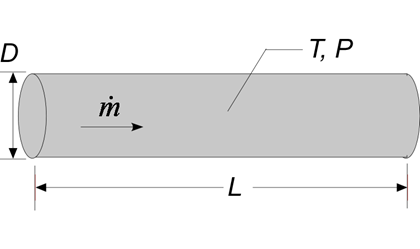Contents - Index

PipeFlowProcedure PipeFlow( Fluid\$, T, P, m_dot, D, L,RelRough: h_T, h_H, DELTAP, Nusselt_T,  f, Re) returns lower and upper bounds on the average heat transfer coefficient and the pressure drop for a specified mass flow rate (m_dot) through a circular tube of diameter D and length L.  The procedure assumes simultaneous hydrodynamic and thermally developing flow as reported in Section 5.2.3 of Nellis and Klein.  Properties are evaluated at the bulk temperature T and pressure P, for all built-in fluids except brines, for which the concentration in mixtures with water is provided in % in place of pressure.  Units of the inputs and outputs depend upon the unit settings in EES.

Inputs:

Fluid\$ can be any fluid in the EES database.  The fluid can be an ideal gas, a real fluid, a brine, or a fluid from the Solid-Liquid property library.

T - the bulk temperature of the fluid  in  [C], [K], [F], or [R].

P -  pressure can be in [Pa], [kPa], [bar], [MPa], [atm], or [psia].  (Note that for brines, this parameter provides the concentration in %)

m_dot  -  mass flow rate in [kg/s], or [lbm/hr]

D -  diameter of the tube in [m] or [ft]

L - length of the tube in [m] or [ft]

RelRough - the ratio of the dispersions on the wall of the tube to the tube diameter (must be between 0 and 0.05)

Outputs: (note that it is not necessary to provide all of the outputs.  See below.)

h_T -  heat transfer coefficient in [W/m^2-K] or [Btu/hr-ft^2-R] assuming that the pipe wall is at constant temperature (lower bound)

h_H -  heat transfer coefficient in [W/m^2-K] or [Btu/hr-ft^2-R] assuming a constant heat flux at the pipe wall (upper bound)

DELTAP - pressure difference between the inlet and outlet of the pipe in the pressure units set in the EES Unit System dialog

Nusselt_T - Nusselt number (determined for a constant temperature wall) [-]

f -  friction factor [-]

Re - Reynolds number [-]

Notes

This procedure  calculates the Reynolds and Prandtl numbers and then calls PipeFlow_N.

Two values of the heat transfer coefficient are returned.  The first, h_T is determined assuming that the wall is at constant temperature.  The second is determined assuming that the wall is subjected to a constant heat flux.  For laminar flow, these values should provide lower and upper bounds on the heat transfer coefficient.  For turbulent flow, these values are identical.

The procedure will determine if the flow is laminar or turbulent.  Transitional flow is assumed to occur for Reynold's numbers between 2300 and 3000 and interpolation is applied between the laminar and turbulent correlations.  The ratio of L/D is used to apply a developing flow correction based on simultaneous hydrodynamic and thermal development; set L to a large number if developing flow corrections are not applicable.

Inputs and outputs in the Call statement are separated with a list separator character, which is a comma in the US system and a semicolon in the European system.

Example 1:

\$UnitSystem SI K Pa J

T=363 [K]  "average temperature of fluid in pipe"

P=101300 [Pa] "pressure of air in pipe"

m_dot=0.05 [kg/s]                 "flow rate"

D=0.15 [m]                              "pipe diameter"

L=500 [m]                                 "pipe length"

RelRough=0                           "relative roughness"

Call pipeflow('Air', T, P, m_dot, D, L,RelRough: h_T, h_H, DELTAP, Nusselt_T,  f, Re)

{Solution:

DELTAP=357 [Pa]

f=0.02601 [-]

h_H=10.32 [W/m^2-K]

h_T=10.32 [W/m^2-K]

Nusselt_T=51.21 [-]

Re=19,851 [-]

}

NOTE:  Starting with version 9.513, it is not necessary to supply all of the outputs to a Procedure.  If, for example, you only want to return output h_H, the call statement can appear as:

Call pipeflow('Air', T, P, m_dot, D, L,RelRough: {h_T}, h_H, {DELTAP}, {Nusselt_T}, {f}, {Re}){The quantities in braces are optional, but the list separators are required.}

Example 2:

\$unitSystem SI Pa K J

T=363 [K] "average temperature of fluid in duct"

C=50[%] "concentration of the propylene glycol-water solution"

m_dot=0.10 [kg/s]   "flow rate"

D=0.025 [m] "inner pipe diameter"

L=5 [m]  "length"

RelRough=0.005 [-] "relative roughness"

Call pipeflow('PG',T,C,m_dot,D,L,RelRough:h_T, h_H ,DELTAP, Nusselt_T, f, Re)

{Solution:

DELTAP=179.2 [Pa]

f=0.04263

h_H=857.9 [W/m^2-K]

Nusselt_T=54.09

Re=5623}